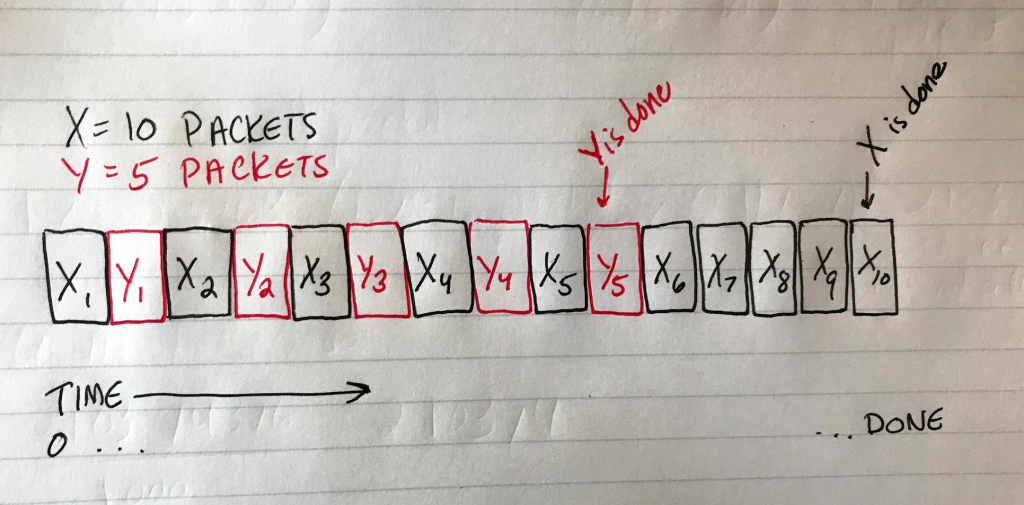# Networking – how to solve the “fileX and fileY” problem

In this post: A detailed, step-by-step guide demonstrating the steps I use to solve a problem similar to one encountered in my CS 372 Intro to Networking class.

You’re given a link with a maximum transmission rate of 70.5 Mbps. Two computers, X and Y, want to transmit starting at the same time. Computer X sends File X (18 MiB) and computer Y sends File Y (130 KiB), both starting at time t = 0.

• Statistical multiplexing is used, with details as follows
• Packet Payload Size = 1000 Bytes
• Ignore Processing and Queueing delays
• Assume partial packets (packets consisting of less than 1000 Bytes of data) are padded so that they are the same size as full packets.
• Assume continuous alternating-packet transmission.
• Computer X gets the transmission medium first.

At what time (t = ?) would File X finish transmitting?

Suppose they want the answer in seconds (hah – not milliseconds like usual!) and they want it rounded to two decimal places.

The “twist” of this problem is that File X and File Y take turns being sent in little chunks of 1000 bytes (plus 24 bytes of overhead).

First a piece of X goes, then a piece of Y, then back to X, etc.

Y is smaller so you run out of Y before you run out of X, but fortunately for us, this problem wants to know when X is done being sent. So really what we’re looking for is how long it takes to send both X and Y.

Here’s a drawing I made to visualize the problem (obviously, X and Y are both way more than just 10 and 5 packets in our real problem).Diagram depicting X1, Y1, X2, Y2, X3, Y3, X4, Y4, X5, Y5, X6, X7, X8, X9, X10 interwoven with each other. Each block represents a packet.

Step 1: Figure out how many packets have to be sent, total

Our packet size (1000 bytes) and our overhead size (24 Bytes) are given in bytes, so I’m going to turn the two filesizes into Bytes instead of bits for this one. (We can always turn it into bits later if needed.)

```File X = 18 MiB // turn to bytes by multiplying by 1024 twice
= 18 * 1024 * 1024
= 18874368 bytes```
```File Y = 130 KiB // turn to bytes by multiplying by 1024 once
= 130 * 1024
= 133120 bytes```

Now X and Y are in the same units (bytes) and we can use that to figure out how many packets of 1000 bytes each one would be divided into.

`File X = 18874368 Bytes / 1000 = 18874.368 packets`

Our result isn’t a whole number. What should we do with the “partial packet”? Well, the problem tells us that any partial packets should be padded up to a full size. In practice, this just means we round up to the next nearest whole number. 18874.368 becomes 18875 packets for File X.

Now do the same for File Y:

```File Y = 133120 Bytes / 1000 = 133.12
Round up to get 134 packets for File Y```

(Save these numbers for later, we’ll come back to them.)

Step 2: Calculate the time needed to send one packet plus its overhead

Add the packet size and the overhead size (both must be in the same units; here, that’s bytes but your variation of this problem may differ). Since transmission rate is in bits, let’s also multiply the packet size + overhead size by 8 to put it in bits, and multiply the transmission rate by 10^6 to put it in bits also. That looks like…

```L/R, where R's units is what the outcome of this calculation will be
= (1000 Bytes + 24 Bytes * 8) / (70.5 Mbps x 10^6)
= (8192) / (70.5 x 10^6)
= 0.0001161985816 seconds // same units as R (bits per second)
= 0.11619858156 msec // multiply by 1000 to get milliseconds```

We now know that it takes 0.11619858156 milliseconds to send one packet (and its overhead). Save this number for later.

Step 3: Calculate the time it takes to send all packets

This step is easy: we know how many packets we have between File X and File Y, and we know how long it takes to send one of them, so let’s figure out how long it takes to send all of them.

`18875 packets + 134 packets = 19009 packets to send`
`19009 packets x 0.11619868156 msec = 2208.820738 milliseconds to send all `

Step 4: Convert to seconds and round to 2 decimal places to get the final answer

This variation of the problem wants the solution in seconds, so divide our result from Step 3 by 1000 to get 2.208820738 seconds and round it to two decimal places to get 2.21 seconds, which is when time at which File X will be done sending.

What if it wanted to know when the smaller file was done sending?

Well, let’s look at our diagram again. Here we can see that for every packet of Y sent, a packet of X has already been sent.For every packet of Y that is sent, one from X precedes it. In other words, to send all 5 packets of Y we must also send 5 packets of X.

We know Y is made up of 134 packets, so that many packets of X will also be sent. That means for our final answer all we have to do is figure out how long it’ll take to send 134*2 packets.

```134 * 2 = 268 // all of Y + same amount of X
268 packets x 0.11619868156 msec = 31.14124666 msec to send File Y```

Convert that answer to seconds and there we have it: 0.03 seconds have elapsed when File Y (the smaller file) is done sending.

This site uses Akismet to reduce spam. Learn how your comment data is processed.